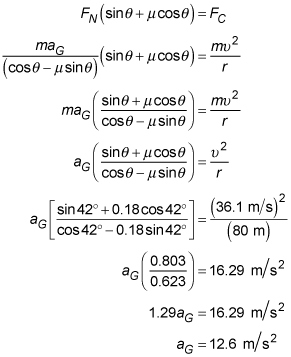##### Physics I: 501 Practice Problems For Dummies (+ Free Online Practice)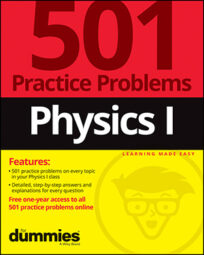Using physics, you can see how normal force and gravity act on an object as it moves around a curve. This is useful if, for example, you want to find the maximum allowable speed of a car before it starts to slip off a curved road.

Here are some practice questions that you can try.

## Practice questions

1. What is the critical speed that a 1,500-kilogram car must maintain to avoid slipping while driving along a section of frictionless road with a radius of curvature of 25 meters and an inclination of 10 degrees?

2. Trial runs along a racetrack located on a mysterious planet have shown that a maximum speed of 130 kilometers per hour can be maintained around the 80-meter-radius turns if those turns are banked at 42 degrees. What is the magnitude of the gravitational acceleration on this planet's surface if the coefficient of friction between a vehicle's tires and the track is 0.18?

Round your answer to the nearest tenth of a meter per second squared.

The following are the answers to the practice questions:

1. 7 m/s

Start by drawing the forces acting on the car. No friction is present, so the only two forces are the force of gravity, pulling straight down, and the normal force. Make sure to break your normal force into its components so that you can sum forces in both the horizontal direction and the vertical direction.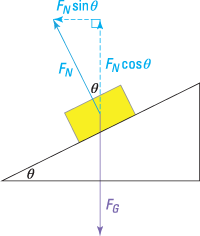Because the car is not accelerating upward or downward, the sum of the vertical forces must equal 0: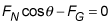Knowing that the force of gravity near Earth's surface is equal to mg, you can rewrite this equation and rearrange it to solve for the unknown normal force: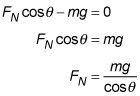The sum of the horizontal forces provides the centripetal force keeping the car in its circular motion: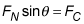Now use the result you obtained earlier for the normal force and the centripetal force equation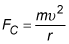(where m is the mass of the object,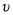is the object's velocity, and r is the radius of the curve the object is traversing) to solve the equation.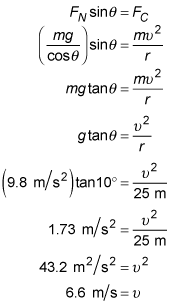Then round to the requested amount: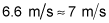2. 12.6 m/s2

Start by drawing the forces acting on the vehicle. Three forces are involved in this situation: the force of gravity, pulling straight down; the normal force, or the force with which the ground is pushing against the vehicle, directed perpendicularly away from the ground; and the force of friction, directed in the opposite direction of the vehicle's motion. Speeding along, the vehicle prefers to keep going "straight," which means heading "up" the bank, so you need to draw your friction force heading "down" the bank. Make sure to break your normal and friction forces into their components so that you can sum forces in the horizontal direction and in the vertical direction.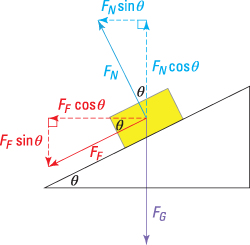Because the vehicle is not accelerating upward or downward, the sum of the vertical forces must equal 0: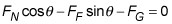Knowing that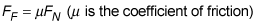and that the force of gravity is the product of mass and the acceleration due to gravity (aG), you can rewrite this equation and rearrange it to solve for the unknown normal force: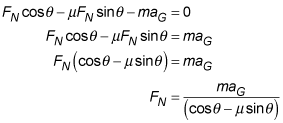The sum of the horizontal forces provides the centripetal force keeping the vehicle in its circular motion: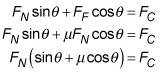Before jumping into the calculations, make sure you convert the velocity to "correct" units: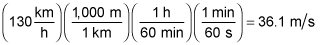Now use the result you obtained earlier for the normal force and the centripetal force equation: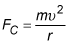where m is the mass of the object,is the object's velocity, and r is the radius of the curve the object is traversing, to solve the equation.